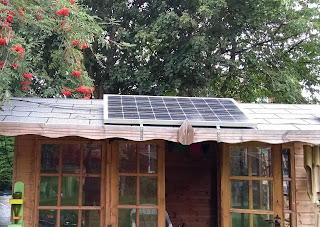## Friday, 13 September 2019

### Watts, Volts, Amperes, Ampere hours and Watt hours - what does it all mean?

When planning your off-grid Solar Power system it helps to understand the numbers and terminology. This post explains in simple terms the meaning of these and how they relate to each other.

Watts - This is a measure of how much power an appliance takes. The more power an appliance takes the quicker it will drain the battery. Some examples are given below for a typical leisure battery of 100 Ah capacity (based on discharge down to 50%)

LED light bulb    5 Watts         5 days
TV                       40 Watts       15 hours
Kettle                   2000 Watts   30 minutes  (only theoretical as the battery cannot supply this power)

Note: 1000 Watts = 1 kilo Watt (or 1 kW)

Volts - The higher the voltage the less current is required to power an appliance. Most batteries used for solar systems start off at 12 V. If you put 2 together in series you get 24 V. When powering an appliance rated at 300 Watts this will reduce the current from 25 Amps to 12.5 Amps. This in turn will reduce power wasted in the cabling and also allow the use of thinner cables.

Note: Mains voltage is 230 V AC in Europe and 110 V AC in the US

Amperes - Higher powered appliances take more current which is measured in Amperes. This is often shorted to Amps. The higher the current the faster the battery discharges. Here are some examples when using a 12 V battery.

LED light bulb      5 Watts        0.4 Amps
TV                         40 Watts      3.3 Amps
Kettle                    2000 Watts  166 Amps

Note: Volts x Amperes = Watts

Amp hours - This is often shortened to Ah. This is used to show the capacity of a battery. So a battery rated as 100 Ah is capable of supplying 1 Amp for 100 hours or 2 Amps for 50 hours. (in practice its best to only use 50% of a battery to avoid shortening its life).

To increase battery capacity, many batteries can be connected in parallel. eg 5 batteries of 100 Ah capacity in parallel will give a capacity of 500 Ah.

Note: Two batteries can be connected in series to create a 24 V circuit

Watt hours - This is a measure of the total energy stored in a battery as it takes voltage into account. So for a 12 V 100 Ah battery has a total energy of 12 x 100 = 1200 Watt hours (or 600 Watt hours at 50% of capacity).

Two 12 V batteries in series will give a 24 V system with 100 Ah capacity resulting in a total energy of 2400 Watt hours.

Earlier we showed how long a TV could be used for using a 100 Ah battery. This was worked out dividing 600 (Watt hours) by 40 Watts (the power taken by the TV) which gives 15 hours.

Solar Power system - Lights required for shed at the end of the garden

We need to power 2 x 10 Watt LED lights fitted with motion detectors. Total power is 20 Watts. A 12 V battery of 20 Ah capacity will have a total energy of 240 Watt hours. Assuming that we use 50% of the capacity (120 Watt hours) this battery will power the 2 lamps for a total of 6 hours continuously.

As the lamps are only occasionally triggered we can estimate that they are on for a total of 30 minutes each night giving us 12 days of operation without any sunshine. If we use a 20 Watt solar panel and assume that in winter it produces 10 Watts over 4 hours every day then over 3 days it should fully charge the battery.

So a 20 Watt solar panel and a 20 Ah battery should give us a reliable Solar Power system to provide half an hour of lighting in the shed at the bottom of the garden.

To monitor power usage a Watt Meter is always very useful. It shows Watts, Amps, Volts and Watt hours. Buy from Amazon

Solar Power system - Power for off-grid holiday home

We need to power LED lights and TV at holiday home for the weekend as well charging the phones. All the lights have a total power of 30 Watts and need to be on for 5 hours every day. The TV (40 Watts) is used for 3 hours a day. The power required to charge phones is negligible.

LED lights need a total energy of 30 x 5 x 2 = 300 Watt hours
The TV needs a total energy of 40 x 3 x 2 = 240 Watt hours

So the battery energy will have to be a total of 540 x 2 = 1080 Watt hours as we only want to discharge the battery down to 50% of its capacity. Using a 12 V battery the capacity will have to be at least  90 Ah. This is arrived at by dividing 1080 by 12.

As the property is not occupied during the week, only a small solar panel is required to charge up the battery - ready for the next weekend.

Summary

This post presents a basic guide to the terminology used when planning a stand alone Solar Power system.